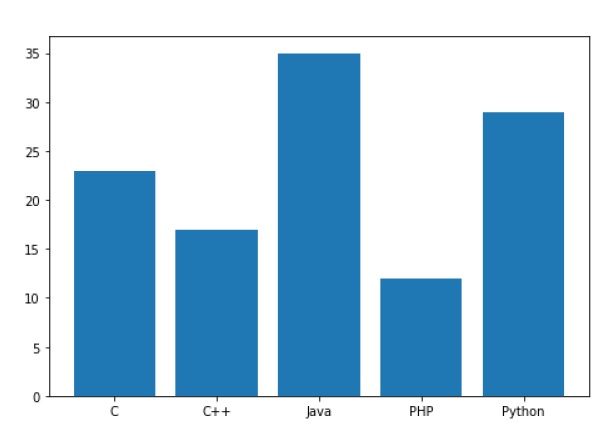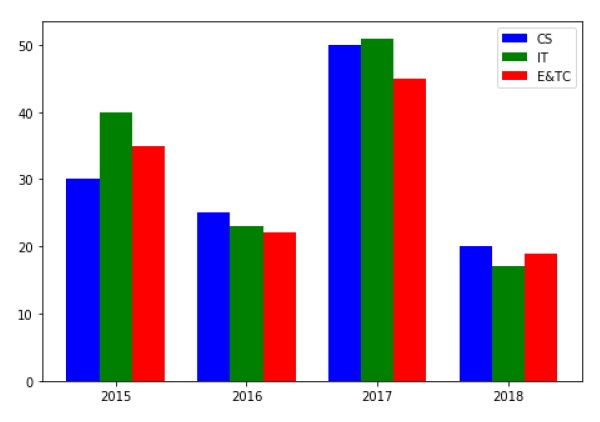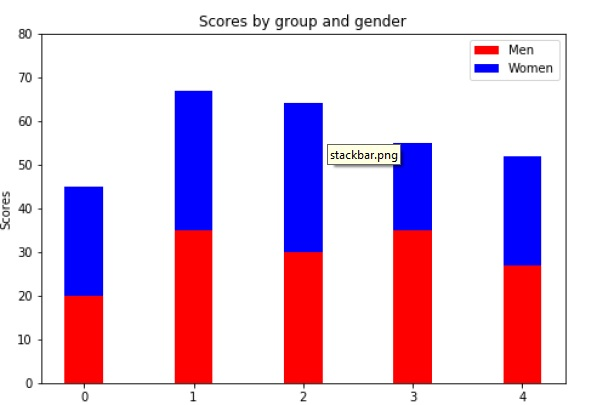# Matplotlib - Bar Plot

A bar chart or bar graph is a chart or graph that presents categorical data with rectangular bars with heights or lengths proportional to the values that they represent. The bars can be plotted vertically or horizontally.

A bar graph shows comparisons among discrete categories. One axis of the chart shows the specific categories being compared, and the other axis represents a measured value.

Matplotlib API provides the bar() function that can be used in the MATLAB style use as well as object oriented API. The signature of bar() function to be used with axes object is as follows −

```ax.bar(x, height, width, bottom, align)
```

The function makes a bar plot with the bound rectangle of size (x −width = 2; x + width=2; bottom; bottom + height).

The parameters to the function are −

 x sequence of scalars representing the x coordinates of the bars. align controls if x is the bar center (default) or left edge. height scalar or sequence of scalars representing the height(s) of the bars. width scalar or array-like, optional. the width(s) of the bars default 0.8 bottom scalar or array-like, optional. the y coordinate(s) of the bars default None. align {‘center’, ‘edge’}, optional, default ‘center’

The function returns a Matplotlib container object with all bars.

Following is a simple example of the Matplotlib bar plot. It shows the number of students enrolled for various courses offered at an institute.

```import matplotlib.pyplot as plt
fig = plt.figure()
langs = ['C', 'C++', 'Java', 'Python', 'PHP']
students = [23,17,35,29,12]
ax.bar(langs,students)
plt.show()
```When comparing several quantities and when changing one variable, we might want a bar chart where we have bars of one color for one quantity value.

We can plot multiple bar charts by playing with the thickness and the positions of the bars. The data variable contains three series of four values. The following script will show three bar charts of four bars. The bars will have a thickness of 0.25 units. Each bar chart will be shifted 0.25 units from the previous one. The data object is a multidict containing number of students passed in three branches of an engineering college over the last four years.

```import numpy as np
import matplotlib.pyplot as plt
data = [[30, 25, 50, 20],
[40, 23, 51, 17],
[35, 22, 45, 19]]
X = np.arange(4)
fig = plt.figure()
ax.bar(X + 0.00, data, color = 'b', width = 0.25)
ax.bar(X + 0.25, data, color = 'g', width = 0.25)
ax.bar(X + 0.50, data, color = 'r', width = 0.25)
```The stacked bar chart stacks bars that represent different groups on top of each other. The height of the resulting bar shows the combined result of the groups.

The optional bottom parameter of the pyplot.bar() function allows you to specify a starting value for a bar. Instead of running from zero to a value, it will go from the bottom to the value. The first call to pyplot.bar() plots the blue bars. The second call to pyplot.bar() plots the red bars, with the bottom of the blue bars being at the top of the red bars.

```import numpy as np
import matplotlib.pyplot as plt
N = 5
menMeans = (20, 35, 30, 35, 27)
womenMeans = (25, 32, 34, 20, 25)
ind = np.arange(N) # the x locations for the groups
width = 0.35
fig = plt.figure()
ax.bar(ind, menMeans, width, color='r')
ax.bar(ind, womenMeans, width,bottom=menMeans, color='b')
ax.set_ylabel('Scores')
ax.set_title('Scores by group and gender')
ax.set_xticks(ind, ('G1', 'G2', 'G3', 'G4', 'G5'))
ax.set_yticks(np.arange(0, 81, 10))
ax.legend(labels=['Men', 'Women'])
plt.show()
```